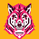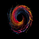2928 visitas
2928
This version of the MACD indicator is 'trawling' (checking) if the MACD histogram and the zero line crossing with the MACD line are both positive or negative. The idea behind this is to show areas with higher or lower risk.

Features:
1. Enable the bar color
2. Enable the background color
3. Change zero line value

FYI:

"The MACD-Histogram is an indicator of an indicator. In fact, MACD is also an indicator of an indicator. This means that the MACD-Histogram is the fourth derivative of price."

First derivative: 12-day EMA and 26-day EMA
Second derivative: MACD (12-day EMA less the 26-day EMA )
Third derivative: MACD signal line (9-day EMA of MACD )
Fourth derivative: MACD-Histogram ( MACD less MACD signal line)

Source: http://stockcharts.com/school/doku.php?s...
```study(title="MACD Color Trawler (by ChartArt)", shorttitle="CA_-_MACD_CT")

// Version 1.0
// Idea by ChartArt on May 12, 2015.
//
// The indicator is 'trawling' (checking) if the MACD histogram
// and the zero line crossing with the MACD line
// are both positive or negative.
//
// List of my work:

source = close
fastLength = input(12, minval=1), slowLength=input(26,minval=1)
signalLength=input(9,minval=1)
fastMA = ema(source, fastLength)
slowMA = ema(source, slowLength)
macd = fastMA - slowMA
signal = sma(macd, signalLength)
hist = macd - signal

switch1=input(true, title="Enable Bar Color?")
switch2=input(true, title="Enable Background Color?")

plot(macd, color=blue,linewidth=2)
plot(signal, color=gray,linewidth=1)

// Histogram Color
GetHistogramColor =	iff(hist > 0, 1,
iff(hist < 0, -1, nz(GetHistogramColor, 0)))
ColorHistogram = GetHistogramColor == -1 ? red: GetHistogramColor == 1 ? green : blue
plot(hist, color=ColorHistogram, style=histogram,linewidth=4)

// Bar Color
Trigger = input(0, title="Zeroline Trigger Value?")
GetBarColor =	iff((macd > Trigger) and (hist > 0), 1,
iff((macd < Trigger) and (hist < 0), -1, nz(GetBarColor, 0)))
SelectBarColor = GetBarColor == -1 ? red: GetBarColor == 1 ? green: blue
barcolor(switch1?SelectBarColor:na)

// Background Color
GetBackgroundColor =	iff((macd > Trigger) and (hist > 0), 1,
iff((macd < Trigger) and (hist < 0), -1, nz(GetBackgroundColor, 0)))
SelectBackgroundColor = GetBackgroundColor == -1 ? red: GetBackgroundColor == 1 ? green: blue
bgcolor(switch2?SelectBackgroundColor:na, transp=90)

```Hi, great script, much appreciated. Is there any way to set an alert for the background color changes? Can you also please explain what the background color change is based on? Thank you!
Responderj.harrison